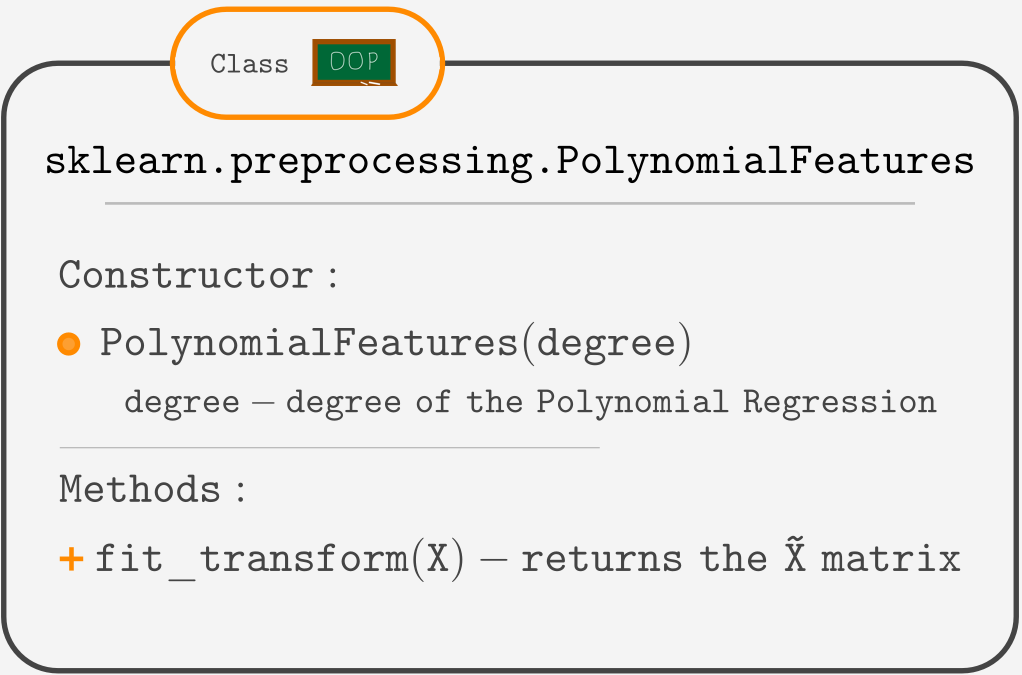Course Content

# Linear Regression with Python

Linear Regression with Python

##Challenge

In this challenge, you are given the good old housing dataset, but this time only with the 'age' feature.Let's build a scatterplot of this data.Fitting a straight line to this data may not be a great choice. The price gets higher for either brand-new or really old houses. Fitting a parabola looks like a better choice. And that's what you will do in this challenge.

But before you start, recall the `PolynomialFeatures` class.The `fit_transform(X)` method requires `X` to be a 2-D array (or a DataFrame).
Using `X = df[['column_name']]` will get your `X` suited for `fit_transform()`.
And if you have a 1-D array, use `.reshape(-1, 1)` to make a 2-D array with the same contents.

The task is to build a Polynomial Regression of degree 2 using `PolynomialFeatures` and `OLS`.1. Assign the `X` variable to a DataFrame containing column `'age'`.
2. Create an `X_tilde` matrix using the `PolynomialFeatures` class.
5. Reshape `X_new` to be a 2-D array.
6. Preporcess `X_new` the same way as `X`.